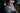# Linear Regression with TensorFlow.js

###Eric Jinks — June 2nd, 2018

Recently, Google released TensorFlow.js which is JavaScript (browser & node) version of the open source machine learning framework, TensorFlow.

TensorFlow.js allows us to build, train and deploy ML models in the browser. Existing models compiled with TensorFlow or Keras can be converted and imported by TensorFlow.js, ready for inference or further retraining of the model.

To get my head around the API, I created a “hello world” linear regression model. In the canvas below, as you click to add points, the linear regression model will be fit to the new data, visualised by the prediction line (using Two.js).

### Variables

As the points are added to the canvas, our model will attempt to learn the coefficients m and b of the function: y = mx + b. We initialise each with a random number.

Note that we cannot use plain JS numbers, we must use `tf.scalar`.

``````// Our coefficient variables
const m = tf.variable(tf.scalar(Math.random())) // slope
const b = tf.variable(tf.scalar(Math.random())) // y intercept``````

### Predict function

We create the linear equation using TensorFlow.js math operations. The `tf.tidy()` function will clear any tensors we create inside this function to avoid memory leaks.

``````const predict = x =>
tf.tidy(() => {
// Create a vector of x values
const xVector = tf.tensor1d(x)
// y = mx + b
return yPred
})``````

### Training the model

With the initial random coefficient values, our prediction line will not fit our data at all. To train the model to learn ideal values for the coefficients, we will create the following:

• the loss function will measure how well our linear equation fits the data. A lower loss value = closer fit.
• the optimiser function will implement an algorithm that will adjust our coefficient values based on the output of the loss function.
• the train function will iteratively run our optimiser function.
``````// loss function: mean squared error
const loss = (yPred, y) =>
yPred.sub(y).square().mean()

const learningRate = 0.5
const optimizer = tf.train.sgd(learningRate)

// train function: running in the Two.js animation loop ~60 times per second
// optimiser.minimize() automatically adjusts our tf.variable coefficents
const train = () => {
tf.tidy(() => {
if (x_vals.length > 0) {
const y = tf.tensor1d(y_vals)
optimiser.minimize(() => loss(predict(x_vals), y))
}
})
}``````

After each training loop, I have added a line with the predicted y values for given x values at each edge of the canvas.

``````// getting values from tensors is async
predict([-1, 1]).data().then(yVals => {
// plot the Two.js line on the canvas
two.makeLine(
// x1, y1
-1 * width, height * yVals),
// x2, y2
1 * width, height * yVals
})``````

If you want to see more of the source code of this demo, check out the repo on github. This demo is based on Daniel Schiffman's video. Head to the Tensorflow.js docs for more info.